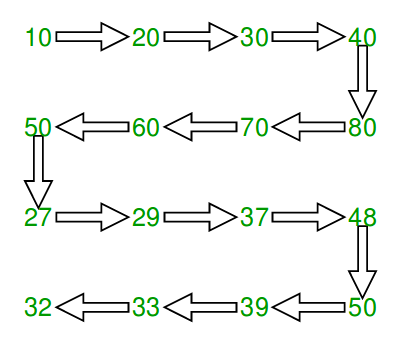Related Articles
Print matrix in snake pattern
• Difficulty Level : Basic
• Last Updated : 14 Aug, 2018

Given an n x n matrix .In the given matrix, you have to print the elements of the matrix in the snake pattern.

Examples :

```Input :mat[][] = { {10, 20, 30, 40},
{15, 25, 35, 45},
{27, 29, 37, 48},
{32, 33, 39, 50}};

Output : 10 20 30 40 45 35 25 15 27 29
37 48 50 39 33 32

Input :mat[][] = { {1, 2, 3},
{4, 5, 6},
{7, 8, 9}};
Output : 1 2 3 6 5 4 7 8 9

```## Recommended: Please solve it on “PRACTICE ” first, before moving on to the solution.

We traverse all rows. For every row, we check if it is even or odd. If even, we print from left to right else print from right to left.

## C++

 `// C++ program to print matrix in snake order``#include ``#define M 4``#define N 4``using` `namespace` `std;`` ` `void` `print(``int` `mat[M][N])``{``    ``// Traverse through all rows``    ``for` `(``int` `i = 0; i < M; i++) {`` ` `        ``// If current row is even, print from``        ``// left to right``        ``if` `(i % 2 == 0) {``            ``for` `(``int` `j = 0; j < N; j++)``                ``cout << mat[i][j] << ``" "``;`` ` `        ``// If current row is odd, print from``        ``// right to left``        ``} ``else` `{``            ``for` `(``int` `j = N - 1; j >= 0; j--)``                ``cout << mat[i][j] << ``" "``;``        ``}``    ``}``}`` ` `// Driver code``int` `main()``{``    ``int` `mat[][] = { { 10, 20, 30, 40 },``                    ``{ 15, 25, 35, 45 },``                    ``{ 27, 29, 37, 48 },``                    ``{ 32, 33, 39, 50 } };`` ` `    ``print(mat);``    ``return` `0;``}`

## Java

 `// Java program to print matrix in snake order``import` `java.util.*;``class` `GFG``{``    ``static` `void` `print(``int` `[][] mat)``    ``{``        ``// Traverse through all rows``        ``for` `(``int` `i = ``0``; i < mat.length; i++)``        ``{`` ` `            ``// If current row is even, print from``            ``// left to right``            ``if` `(i % ``2` `== ``0``)``            ``{``                ``for` `(``int` `j = ``0``; j < mat[``0``].length; j++)``                    ``System.out.print(mat[i][j] +``" "``);`` ` ` ` `            ``// If current row is odd, print from``            ``// right to left``            ``}``            ``else``            ``{``                ``for` `(``int` `j = mat[``0``].length - ``1``; j >= ``0``; j--)``                    ``System.out.print(mat[i][j] +``" "``);``            ``}``        ``}``    ``}`` ` `    ``// Driver code``    ``public` `static` `void` `main(String[] args)``    ``{``        ``int` `mat[][] = ``new` `int``[][]``        ``{``            ``{ ``10``, ``20``, ``30``, ``40` `},``            ``{ ``15``, ``25``, ``35``, ``45` `},``            ``{ ``27``, ``29``, ``37``, ``48` `},``            ``{ ``32``, ``33``, ``39``, ``50` `}``        ``};`` ` `        ``print(mat);``    ``}``}``/* This code is contributed by Mr. Somesh Awasthi */`

## Python 3

 `# Python 3 program to print``# matrix in snake order``M ``=` `4``N ``=` `4`` ` `def` `printf(mat):``    ``global` `M, N``     ` `    ``# Traverse through all rows``    ``for` `i ``in` `range``(M):``         ` `        ``# If current row is``        ``# even, print from``        ``# left to right``        ``if` `i ``%` `2` `=``=` `0``:``            ``for` `j ``in` `range``(N):``                ``print``(``str``(mat[i][j]),``                          ``end ``=` `" "``)`` ` `        ``# If current row is ``        ``# odd, print from``        ``# right to left``        ``else``:``            ``for` `j ``in` `range``(N ``-` `1``, ``-``1``, ``-``1``):``                ``print``(``str``(mat[i][j]), ``                          ``end ``=` `" "``)`` ` `# Driver code``mat ``=` `[[ ``10``, ``20``, ``30``, ``40` `],``       ``[ ``15``, ``25``, ``35``, ``45` `],``       ``[ ``27``, ``29``, ``37``, ``48` `],``       ``[ ``32``, ``33``, ``39``, ``50` `]]`` ` `printf(mat)`` ` `# This code is contributed``# by ChitraNayal`

## C#

 `// C# program to print ``// matrix in snake order``using` `System;``class` `GFG``{``    ``static` `void` `print(``int` `[,]mat)``    ``{``        ``// Traverse through all rows``        ``for` `(``int` `i = 0; ``                 ``i < mat.GetLength(0); i++)``        ``{`` ` `            ``// If current row is ``            ``// even, print from ``            ``// left to right``            ``if` `(i % 2 == 0)``            ``{``                ``for` `(``int` `j = 0; ``                         ``j < mat.GetLength(1); j++)``                    ``Console.Write(mat[i, j] + ``" "``);`` ` ` ` `            ``// If current row is ``            ``// odd, print from ``            ``// right to left``            ``}``            ``else``            ``{``                ``for` `(``int` `j = mat.GetLength(1) - 1; ``                         ``j >= 0; j--)``                    ``Console.Write(mat[i, j] + ``" "``);``            ``}``        ``}``    ``}`` ` `    ``// Driver code``    ``public` `static` `void` `Main()``    ``{``        ``int` `[,]mat = {{ 10, 20, 30, 40 },``                      ``{ 15, 25, 35, 45 },``                      ``{ 27, 29, 37, 48 },``                      ``{ 32, 33, 39, 50 }};`` ` `        ``print(mat);``    ``}``}`` ` `// This code is contributed``// by ChitraNayal`

## PHP

 `= 0; ``\$j``--)``                ``echo` `\$mat``[``\$i``][``\$j``] , ``" "``;``        ``}``    ``}``}`` ` `// Driver code``\$mat` `= ``array``(``array``(10, 20, 30, 40),``             ``array``(15, 25, 35, 45),``             ``array``(27, 29, 37, 48),``             ``array``(32, 33, 39, 50));`` ` `printLN(``\$mat``);`` ` `// This code is contributed by ajit ``?>`

Output :

```10 20 30 40 45 35 25 15 27 29 37 48 50 39 33 32
```

This article is contributed by Rakesh Kumar. If you like GeeksforGeeks and would like to contribute, you can also write an article using contribute.geeksforgeeks.org or mail your article to contribute@geeksforgeeks.org. See your article appearing on the GeeksforGeeks main page and help other Geeks.

Please write comments if you find anything incorrect, or you want to share more information about the topic discussed above.

Attention reader! Don’t stop learning now. Get hold of all the important DSA concepts with the DSA Self Paced Course at a student-friendly price and become industry ready.

My Personal Notes arrow_drop_up